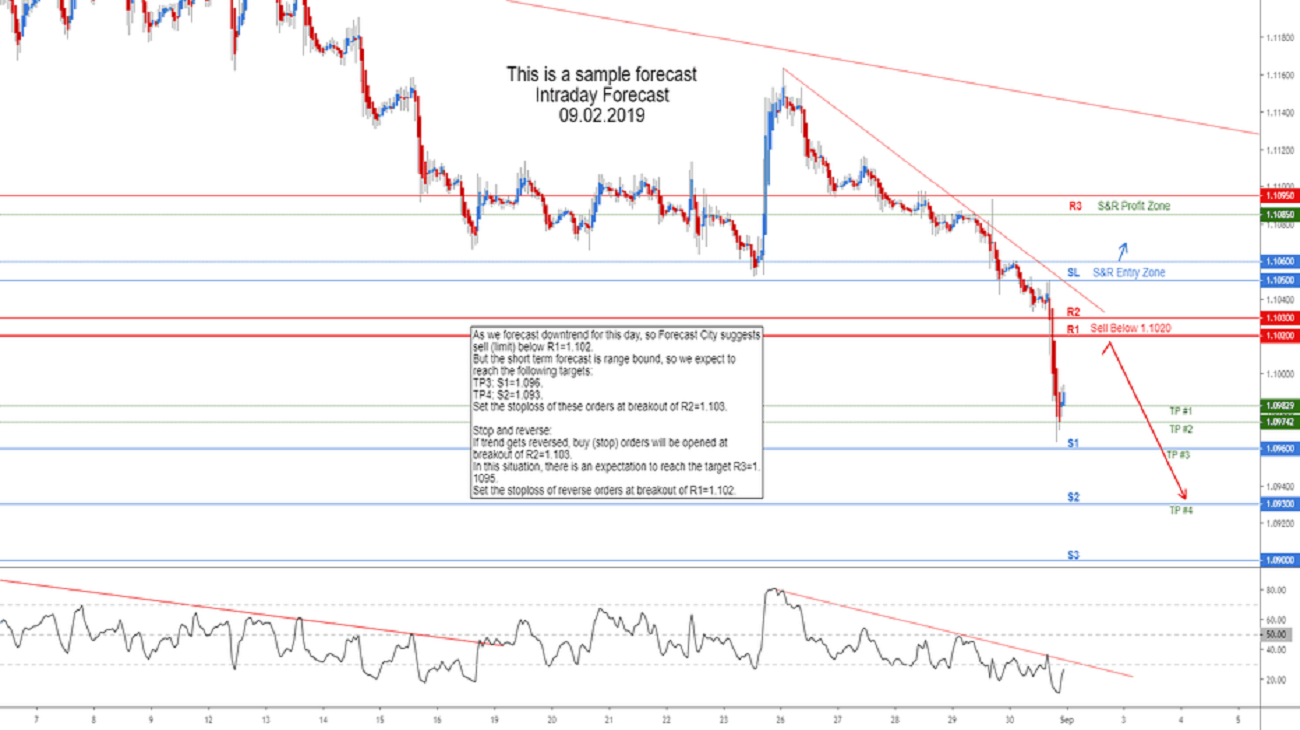As we forecast downtrend for this day, so Forecast City suggests sell (limit) below R1=1.102.
But the short term forecast is range bound, so we expect to reach the following targets:
TP3: S1=1.096.
TP4: S2=1.093.
Set the stoploss of these orders at breakout of R2=1.103.

Stop and reverse:
If trend gets reversed, buy (stop) orders will be opened at breakout of R2=1.103.
In this situation, there is an expectation to reach the target R3=1.1095.
Set the stoploss of reverse orders at breakout of R1=1.102.